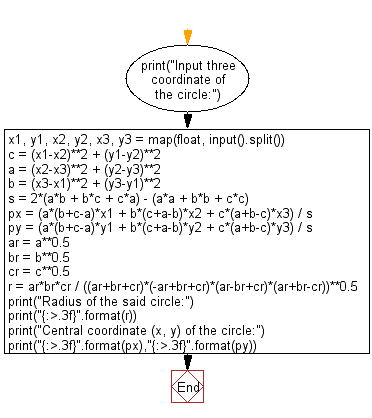﻿ Python: Compute the radius and the central coordinate (x, y) of a circle which is constructed by three given points on the plane surface - w3resource# Python: Compute the radius and the central coordinate (x, y) of a circle which is constructed by three given points on the plane surface

## Python Basic - 1: Exercise-39 with Solution

Write a program to compute the radius and the central coordinate (x, y) of a circle which is constructed by three given points on the plane surface.

Input:
x1, y1, x2, y2, x3, y3 separated by a single space.

Sample Solution:

Python Code:

``````print("Input three coordinate of the circle:")
x1, y1, x2, y2, x3, y3 = map(float, input().split())
c = (x1-x2)**2 + (y1-y2)**2
a = (x2-x3)**2 + (y2-y3)**2
b = (x3-x1)**2 + (y3-y1)**2
s = 2*(a*b + b*c + c*a) - (a*a + b*b + c*c)
px = (a*(b+c-a)*x1 + b*(c+a-b)*x2 + c*(a+b-c)*x3) / s
py = (a*(b+c-a)*y1 + b*(c+a-b)*y2 + c*(a+b-c)*y3) / s
ar = a**0.5
br = b**0.5
cr = c**0.5
r = ar*br*cr / ((ar+br+cr)*(-ar+br+cr)*(ar-br+cr)*(ar+br-cr))**0.5
print("{:>.3f}".format(r))
print("Central coordinate (x, y) of the circle:")
print("{:>.3f}".format(px),"{:>.3f}".format(py))
``````

Sample Output:

```Input three coordinate of the circle:
9 3 6 8 3 6
3.358
Central coordinate (x, y) of the circle:
6.071 4.643
```

Flowchart:Python Code Editor:

Have another way to solve this solution? Contribute your code (and comments) through Disqus.

What is the difficulty level of this exercise?

Test your Programming skills with w3resource's quiz.

﻿

## Python: Tips of the Day

What is the difference between Python's list methods append and extend?

append: Appends object at the end.

```x = [1, 2, 3]
x.append([4, 5])
print (x)
```

Output:

```[1, 2, 3, [4, 5]]
```

extend: Extends list by appending elements from the iterable.

```x = [1, 2, 3]
x.extend([4, 5])
print (x)
```

Output:

```[1, 2, 3, 4, 5]
```

Ref: https://bit.ly/2AZ6ZFq